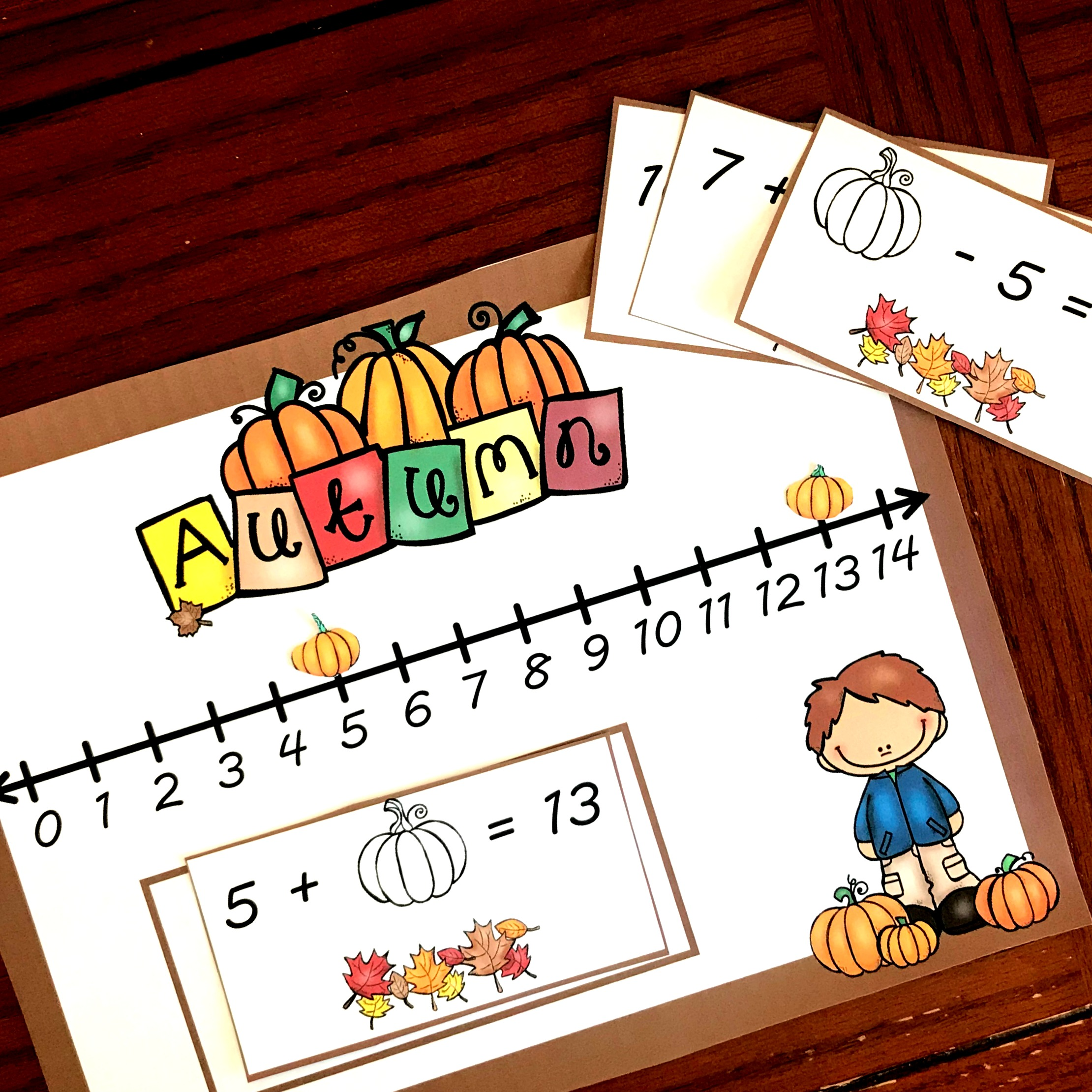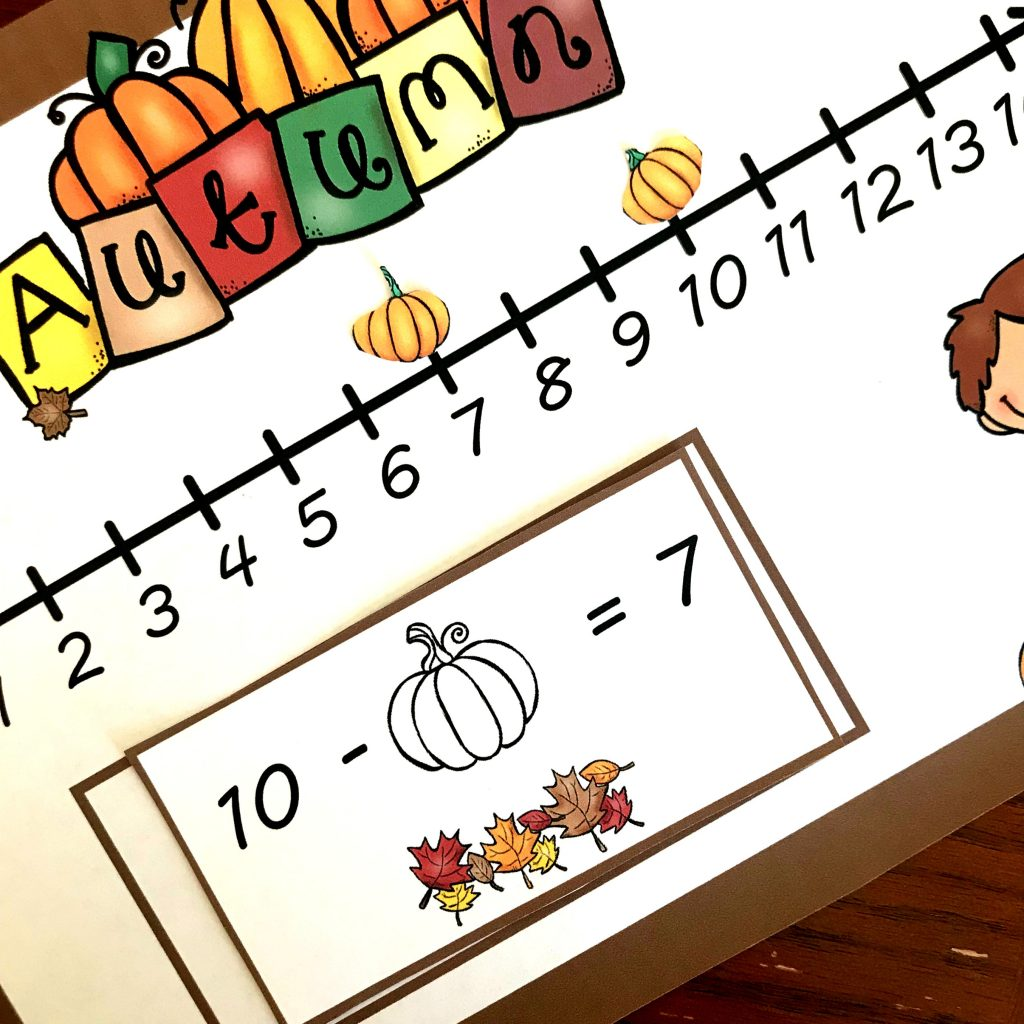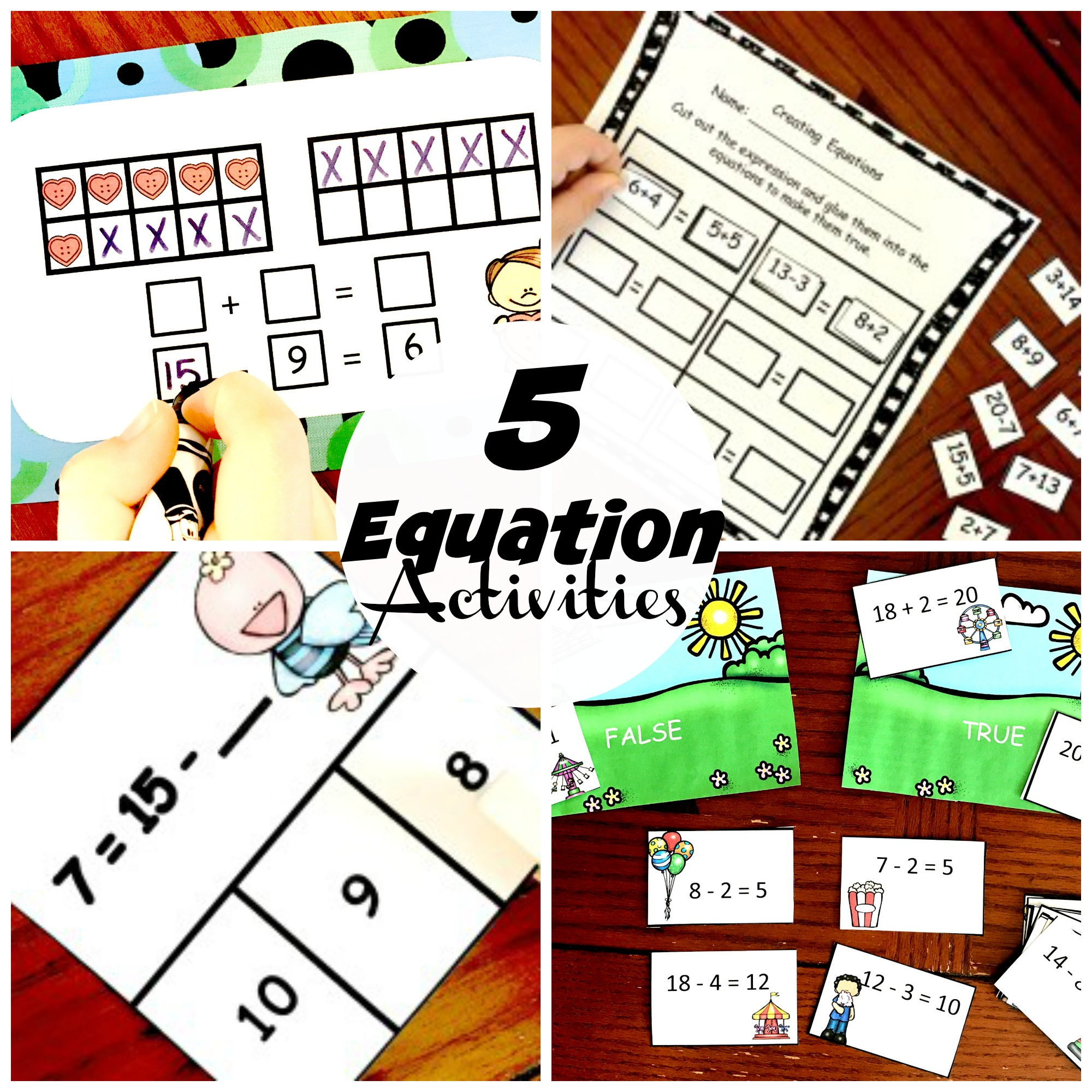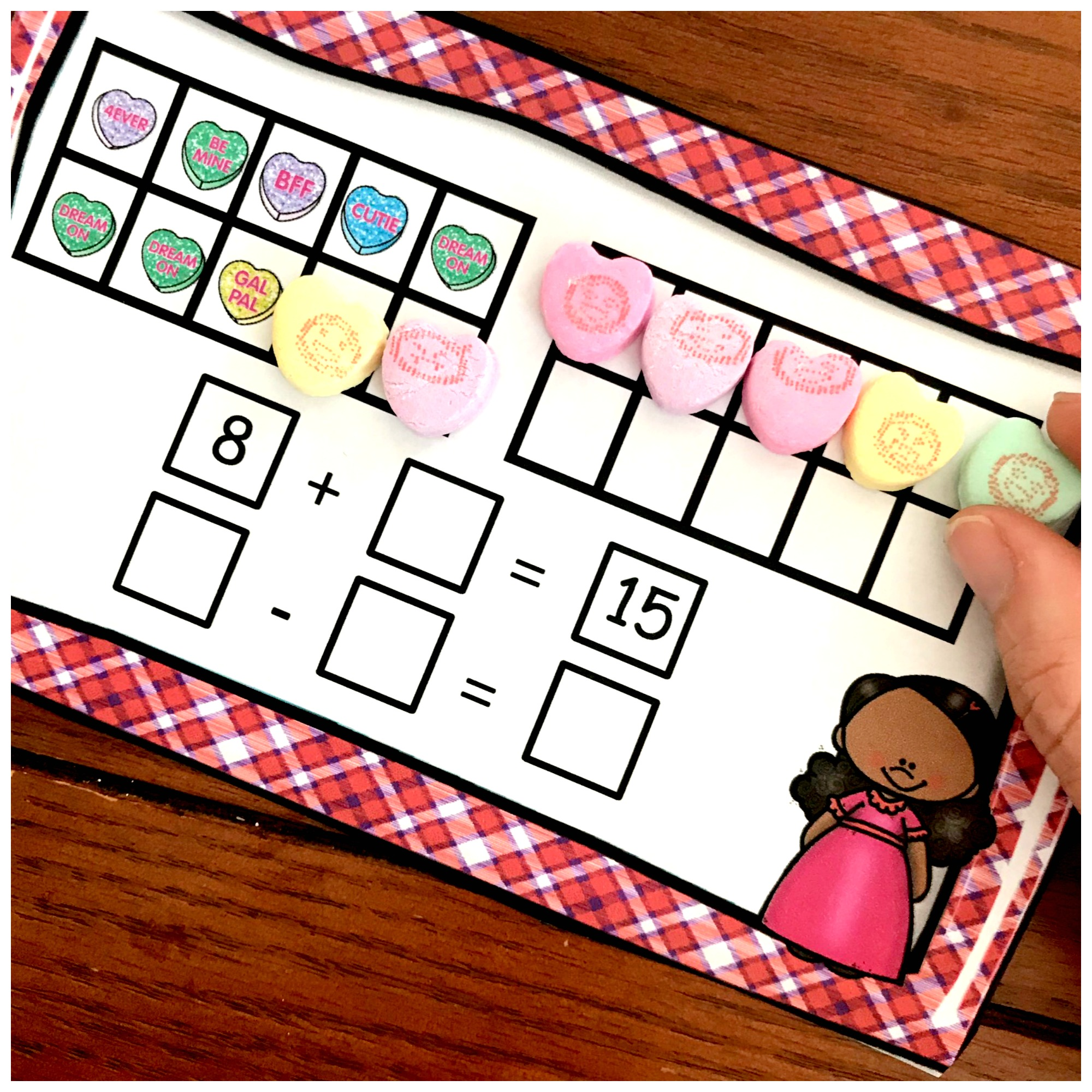Home » Math » FREE Fill in the Missing Number Using A Number Line

# FREE Fill in the Missing Number Using A Number Line

This fill in the missing number activity is a fun way to practice this skill. The children use number lines to find missing addends, subtrahend, or minuend.Some math tasks come easy to my seven year old, and others are a little harder! Filling in missing numbers in equations are definitely one of them.

So I knew a little extra work was needed…..and that is where this fun number line activity came from. Using a number line gave him a concrete way to explore this concept, and help in fill in the missing numbers.

## Prep – Work

1. First, print off all the pages.
2. Next, cut out the equations and pumpkins. (Or you could use magnetic circles.)
3. Now, place magnets on the back of the equations and the pumpkins.
4. Finally, grab a cookie pan and a pencil and you are ready to go.

## Fill in the Missing Numbers Pages:

Now it is time to get down to work.Some of the equations ask the children to find the missing addend, like this one.

And using a number line makes it very easy to find!!!

Begin, by placing a pumpkin on the five and one on the 13. Next, it is time to find out how many jumps there are between the 5 and the 13.

Have the children jump up one number at a time, counting every time they move the pumpkin until they reach the 13. As they do this they will find out that there are eight jumps. so 5 + 8 = 13Next, we move into subtraction…but these problems are very similar to the addition problem. Once again we are trying to find out how many jumps are between the two number.

Start by placing a pumpkin on the 10 and the 7. Since this is subtraction, the children will start at the 10 and see how many jumps it takes to get to the seven.

After three jumps, they will easily see that 10 – 3 = 7This one is a little bit harder. The minuend is missing! So the number our kiddos will be looking for will be bigger than the two numbers they see in the equation.

So, to solve this one we are only going to use one pumpkin. We are going to start at the answer or difference, and place our pumpkin there. Then we will jump up based on the Subtrahend, the number that is to be subtracted.

If we start at one, and jump up five spaces we end up at six.

6 – 5 = 1

Filling in missing numbers can be easy when using number lines!! Enjoy this special fall activity!

You’ve Got This,

Rachel

You may also like:

These activities that have young children exploring expressions and solving for missing numbers and comparing them.Or do some more filling in the missing number activities but using ten frames.